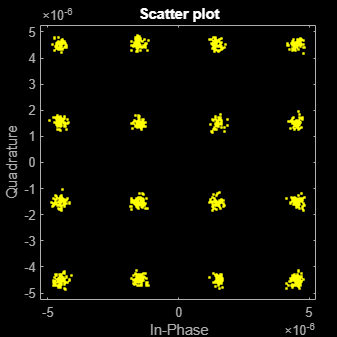Main Content

# comm.ThermalNoise

Add thermal noise to signal

## Description

The `ThermalNoise` object simulates the effects of thermal noise on a complex, baseband signal.

To add thermal noise to a complex, baseband signal:

1. Define and set up your thermal noise object. See Construction.

2. Call `step` to add thermal noise according to the properties of `comm.ThermalNoise`.

Note

Starting in R2016b, instead of using the `step` method to perform the operation defined by the System object™, you can call the object with arguments, as if it were a function. For example, ```y = step(obj,x)``` and `y = obj(x)` perform equivalent operations.

## Construction

`tn = comm.ThermalNoise` creates a receiver thermal noise System object, `H`. This object adds thermal noise to the complex, baseband input signal.

`tn = comm.ThermalNoise(Name,Value)` creates a receiver thermal noise object, `H`, with each specified property set to the specified value. You can specify additional name-value pair arguments in any order as (`Name1`,`Value1`,...,`NameN`,`ValueN`).

## Properties

expand all

Method used to set the noise power, specified as ```'Noise temperature'```, `'Noise figure'`, or ```'Noise factor'```.

Receiver noise temperature, specified in degrees K as a nonnegative real scalar. This property is available when `NoiseMethod` is equal to `'Noise temperature'`. Noise temperature is typically used to characterize satellite receivers because the input noise temperature can vary and is often less than 290 K. Tunable.

Noise figure, specified in dB as a nonnegative real scalar. This property is available when `NoiseMethod` is equal to `'Noise figure'`. Noise figure describes the performance of a receiver and does not include the effect of the antenna. It is defined only for an input noise temperature of 290 K. The noise figure is the dB equivalent of the noise factor. Tunable.

Noise factor, specified as a real scalar greater than or equal to 1. This property is available when `NoiseMethod` is equal to `'Noise factor'`. Noise factor describes the performance of a receiver and does not include the effect of the antenna. It is defined only for an input noise temperature of 290 K. The noise factor is the linear equivalent of the noise figure. Tunable.

Sample rate, specified as in Hz as a positive real scalar. The object computes the variance of the noise added to the input signal as kT×`SampleRate`. The value k is Boltzmann's constant and T is the noise temperature specified explicitly or implicitly via one of the noise methods.

Add 290 K antenna noise to the input signal, specified as a logical scalar. To add 290 K antenna noise, set this property to `true`. This property is available when `NoiseMethod` is equal to `'Noise factor'` or `'Noise figure'`.

The total noise applied to the input signal is the sum of the circuit noise and the antenna noise.

## Methods

 step Add receiver thermal noise
Common to All System Objects
`release`

Allow System object property value changes

## Examples

collapse all

Create a thermal noise object having a noise temperature of 290 K and a sample rate of 5 MHz.

`thNoise = comm.ThermalNoise('NoiseTemperature',290,'SampleRate',5e6);`

Generate QPSK-modulated data having an output power of 20 dBm.

```data = randi([0 3],1000,1); modData = 0.3162*pskmod(data,4,pi/4);```

Attenuate the signal by the free space path loss assuming a 1000 m link distance and a carrier frequency of 2 GHz.

```fsl = (4*pi*1000*2e9/3e8)^2; rxData = modData/sqrt(fsl);```

Add thermal noise to the signal. Plot the noisy constellation.

```noisyData = thNoise(rxData); scatterplot(noisyData)```Create a thermal noise object having a 5 dB noise figure and a 10 MHz sample rate. Specify that the 290 K antenna noise be included.

```thermalNoise = comm.ThermalNoise('NoiseMethod','Noise figure', ... 'NoiseFigure',5, ... 'SampleRate',10e6, ... 'Add290KAntennaNoise',true);```

Generate QPSK-modulated data having a 1 W output power.

```data = randi([0 15],1000,1); modSig = qammod(data,16,'UnitAveragePower',true);```

Attenuate the signal by the free space path loss assuming a 1 km link distance and a 5 GHz carrier frequency.

```fsl = (4*pi*1000*5e9/3e8)^2; rxSig = modSig/sqrt(fsl);```

Add thermal noise to the signal and plot its constellation.

```noisySig = thermalNoise(rxSig); scatterplot(noisySig)```Estimate the SNR.

```mer = comm.MER; snrEst1 = mer(rxSig,noisySig)```
```snrEst1 = 22.6611 ```

Decrease the noise figure to 0 dB, and plot the resultant received signal. Because antenna noise is included, the signal is not completely noiseless.

```thermalNoise.NoiseFigure = 0; noisySig = thermalNoise(rxSig); scatterplot(noisySig)```Estimate the SNR. The SNR is 5 dB higher than in the first case, which is expected given the 5 dB decrease in the noise figure.

`snrEst2 = mer(rxSig,noisySig)`
```snrEst2 = 27.8658 ```
`snrEst2 - snrEst1`
```ans = 5.2047 ```

## Algorithms

Wireless receiver performance is often expressed as a noise factor or figure. The noise factor is defined as the ratio of the input signal-to-noise ratio, Si/Ni to the output signal-to-noise ratio, So/No, such that

`$F=\frac{{S}_{i}/{N}_{i}}{{S}_{o}/{N}_{o}}\text{\hspace{0.17em}}.$`

Given receiver gain G and receiver noise power Nckt, the noise factor can be expressed as

`$\begin{array}{c}F=\frac{{S}_{i}/{N}_{i}}{G{S}_{i}/\left({N}_{ckt}+G{N}_{i}\right)}\\ =\frac{{N}_{ckt}+G{N}_{i}}{G{N}_{i}}\text{\hspace{0.17em}}.\end{array}$`

The IEEE defines the noise factor assuming that noise temperature at the input is T0, where T0 = 290 K. The noise factor is then

`$\begin{array}{c}F=\frac{{N}_{ckt}+G{N}_{i}}{G{N}_{i}}\\ =\frac{GkB{T}_{ckt}+GkB{T}_{0}}{GkB{T}_{0}}\\ =\frac{{T}_{ckt}+{T}_{0}}{{T}_{0}}\text{\hspace{0.17em}}.\end{array}$`

Tckt is the equivalent input noise temperature of the receiver and is expressed as

`${T}_{ckt}={T}_{0}\left(F-1\right)\text{\hspace{0.17em}}.$`

The overall noise temperature of an antenna and receiver, Tsys, is

`${T}_{sys}={T}_{ant}+{T}_{ckt}\text{\hspace{0.17em}},$`

where Tant is the antenna noise temperature.

The noise figure, NF, is the dB equivalent of the noise factor and can be expressed as

`$NF=10{\mathrm{log}}_{10}\left(F\right)\text{\hspace{0.17em}}.$`

## See Also

Introduced in R2012a

## Support

#### Bridging Wireless Communications Design and Testing with MATLAB

Download white paper